## Given problem

Suppose an array of length n sorted in ascending order is rotated between 1 and n times. For example, the array nums = [0,1,2,4,5,6,7] might become:

• `[4,5,6,7,0,1,2]` if it was rotated 4 times.
• `[0,1,2,4,5,6,7]` if it was rotated 7 times.

Notice that rotating an array `[a, a, a, ..., a[n-1]]` 1 time results in the array `[a[n-1], a, a, a, ..., a[n-2]]`.

Given the sorted rotated array nums of `unique` elements, return the minimum element of this array.

You must write an algorithm that runs in `O(log n)` time.

Example 1:

Input: nums = [3,4,5,1,2] Output: 1 Explanation: The original array was [1,2,3,4,5] rotated 3 times. Example 2:

Input: nums = [4,5,6,7,0,1,2] Output: 0 Explanation: The original array was [0,1,2,4,5,6,7] and it was rotated 4 times. Example 3:

Input: nums = [11,13,15,17] Output: 11 Explanation: The original array was [11,13,15,17] and it was rotated 4 times.

Constraints:

• `n == nums.length`
• `1 <= n <= 5000`
• `-5000 <= nums[i] <= 5000`
• All the integers of nums are `unique`.
• nums is sorted and rotated between 1 and n times.

## Some properties of a rotated array

• When we divide a rotated array into two halves, at least one of the two halves will always sorted.

To understand this property, we can see a below image: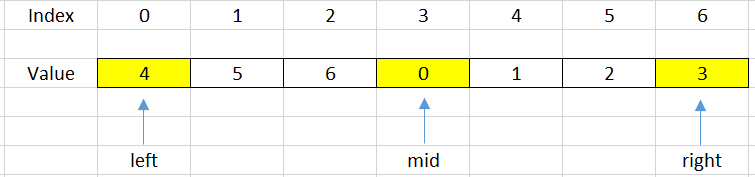If we choose the mid = 3 as the pivot point, we can find that our array is divided into two halves that are increased arrays.

–> So we can apply Binary Search for problems that are relevant to rotated array.

## Solutions for this problem

To solve this problem, we can have two solutions:

• Linear Search

The simple solution to solve it is to use Linear Search. We can scan all elements and compare them with the minimum element.

• Binary Search

Because the a part of array is sorted, so we can use Binary Search to solve it.

``````public static int findMinElement(int[] arr) {
int minPos = 0;
for (int i = 1; i < arr.length - 1; ++i) {
if (arr[minPos] > arr[i]) {
minPos = i;
}
}

return minPos;
}
``````

The complexity of Linear Search:

• Time complexity: O(n) - n is the number of elements of this array
• Space complexity: O(1)

## Using Binary Search algorithm

1. Using Template #1

In a rotated array, we will have some cases that we will cope with when shift it.

• Case 1 - when sorted array is not rotated with any steps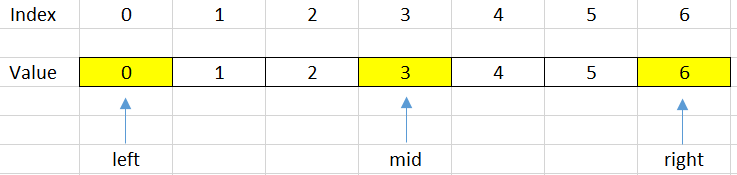In this case, our array is a sorted array but does not rotate with any steps. So, to check this case, we do the below expression:

``````  if (arr[left] <= arr[high]) {
return left;
}
``````
• Case 2 - When our mid index points to the minimum elementSo, we will check it by using the following condition:

``````  int next = (mid + 1) % n;   // n is the length of the array
int prev = (mid + n - 1) % n;

if (arr[mid] < arr[next] && arr[mid] < arr[prev]) {
return mid;
}
``````
• Case 3 - When our mid index points to the element that is belong to the sub-array. It does not contains the minimum element.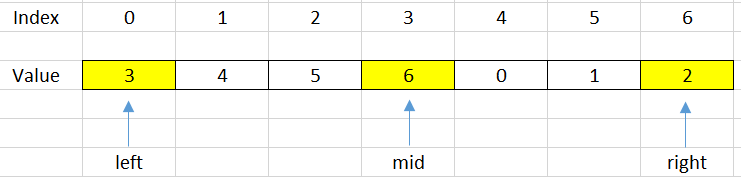In order to know exactly how we are under this case, we will use the below condition:

``````  if (arr[left] <= arr[mid]) {
left = mid + 1;
}
``````

Due to the minimum element that is belong to the other side, so we will shift the right side.

• Case 4 - When our mid index points to the element that is belong to the sub-array. It contains the minimum element.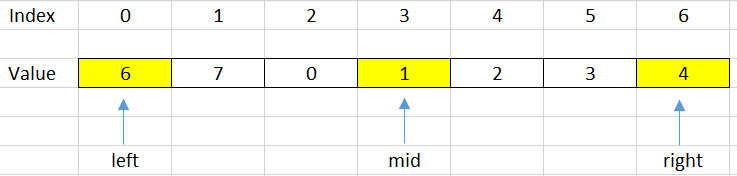``````  if (arr[mid] <= arr[right]) {
right = mid - 1;
}
``````

Based on the four conditions, we will have source code for this problem:

`````` class Solution {
public int findMin(int[] arr) {
int len = arr.length;
int left = 0;
int right = len - 1;

while (left <= right) {
if (arr[left] <= arr[right]) {  // Case 1: sorted array
return arr[left];
}

int mid = left + (right - left) / 2;
int next = (mid + 1) % len;
int prev = (mid + len - 1) % len;
if (arr[mid] <= arr[next] && arr[mid] <= arr[prev]) {   // Case 2: mid index points to the minimum element
return arr[mid];
} else if (arr[mid] <= arr[right]) {    // Case 4
right = mid - 1;
} else if (arr[mid] >= arr[left]) {     // Case 3
left = mid + 1;
}
}

return -1;
}
}
``````
2. Using Template #2

`````` class Solution {
public int findMin(int[] nums) {
if (nums == null) {
return -1;
}

int left = 0;
int right = nums.length - 1;

while (left < right) {
int mid = left + (right - left) / 2;

if (nums[mid] > nums[right]) {
left = mid + 1;
} else {
right = mid;
}
}

return nums[left];
}
}
``````
3. Using Template #3

• Comparing between `mid` and `right` position.

Due to the comparison between `mid` and `right`, the initial value of `right` will be the index of the last element in an array.

``````  int right = nums.length - 1;
``````

The property of a rotated array is that it divides our array into two sorted array in ascending order. So we can choose different points to move `mid`. In this case, we will choose `right` position. In the next way, we will choose `left` position.

Below is some cases that we take care:

• 1st case - No rotation or the number of rotations is equal to the length of the array.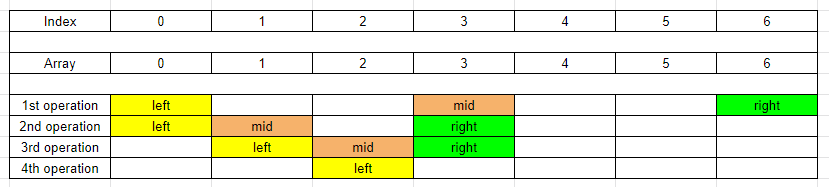• 2nd case - The `mid` points to the minimum element in the array.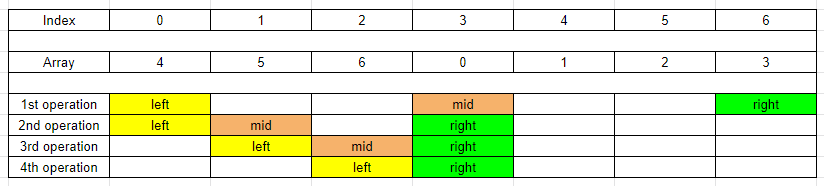We can easily find that it’s the same with the above one.

• 3rd case - The `mid` points to the second child sorted array.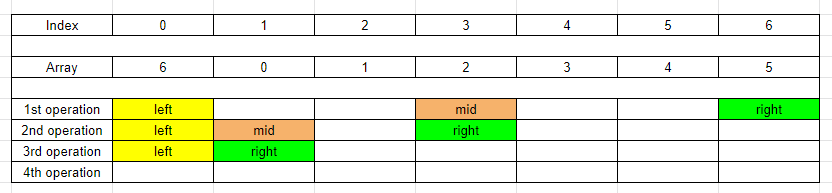• 4th case - The `mid` points to the first child sorted array.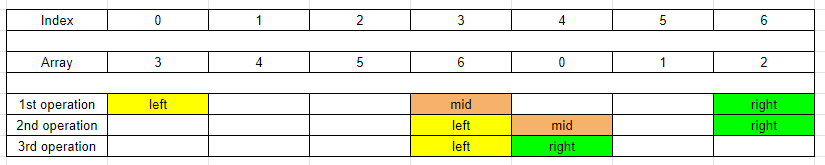Below is our source code.

``````  class Solution {
public int findMin(int[] nums) {
int low = 0;
int high = nums.length - 1;

while (low + 1 < high) {
int mid = low + (high - low) / 2;

if (nums[mid] > nums[high]) {
low = mid;
} else {
high = mid;
}
}

return nums[low] < nums[high] ? nums[low] : nums[high];
}
}
``````
• Comparing between `mid` and `left` position.

``````  public class Solution {
public int findMin(int[] nums) {
int pivot = this.findPivot(nums);
return Math.min(nums, nums[pivot]);
}

private int findPivot(int[] nums) {
int left = 0;
int right = nums.length;

while (left + 1 < right) {
int mid = left + (right - left) / 2;

if (nums[mid - 1] > nums[mid]) {
return mid;
} else if (nums[left] < nums[mid]) {
left = mid;
} else {
right = mid;
}
}

return left;
}
}
``````

The complexity of this solution:

• Time complexity: O(logn)
• Space complexity: O(1)

## Wrapping up

• Understanding how to use some templates of Binary Search algorithm.

• Understanding about what the rotated array is, and its properties.

• When we have two different trends in our array, we can use Binary Search to solve this problem.

• From this problem, we can find that in a rotated array, the index of the minimum element will be equal to the number of rotation of its array.

• Learn how to get the index of the next element in the rotated array by using modulo of the length of the array.

Refer:

153. Find Minimum in Rotated Sorted Array